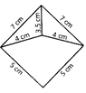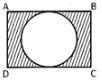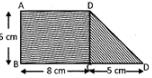Courses

# Test : Area, Perimeter And Volume - 2

## 10 Questions MCQ Test Mathematics for Class 5 (V) - CBSE and NCERT Curriculum | Test : Area, Perimeter And Volume - 2

Description
This mock test of Test : Area, Perimeter And Volume - 2 for Class 5 helps you for every Class 5 entrance exam. This contains 10 Multiple Choice Questions for Class 5 Test : Area, Perimeter And Volume - 2 (mcq) to study with solutions a complete question bank. The solved questions answers in this Test : Area, Perimeter And Volume - 2 quiz give you a good mix of easy questions and tough questions. Class 5 students definitely take this Test : Area, Perimeter And Volume - 2 exercise for a better result in the exam. You can find other Test : Area, Perimeter And Volume - 2 extra questions, long questions & short questions for Class 5 on EduRev as well by searching above.
QUESTION: 1

Solution:
QUESTION: 2

Solution:
QUESTION: 3

### Find the area of the triangle whose base is 15 cm and height is 20 cm.

Solution:
QUESTION: 4

Find the perimeter of the following figure.Solution:

Perimeter is sum of all sides.

QUESTION: 5

Perimeter of a square is 60 cm. Find the area of the square.

Solution:
QUESTION: 6

Look at the following figure:ABCD is a rectangle whose length and breadth are 16 cm and 14 cm respectively. Inside the rectangle ABCD, there is a circle. Find the area of the shaded region.

Solution:
QUESTION: 7

Perimeter of a circle is 1.1 cm. Find the area of the circle.

Solution:
QUESTION: 8

Base of a triangle is 2 cm more than the height. If height of the triangle is 12 cm, find the area of the triangle.

Solution:
QUESTION: 9

Perimeter of a square is equal to the perimeter of the rectangle. If length and breadth of the rectangle is 24 cm and 20 respectively. Find the area of the square.

Solution:
QUESTION: 10

Look at the following figure:ABCD is a rectangle. Find the area of the figure.

Solution: